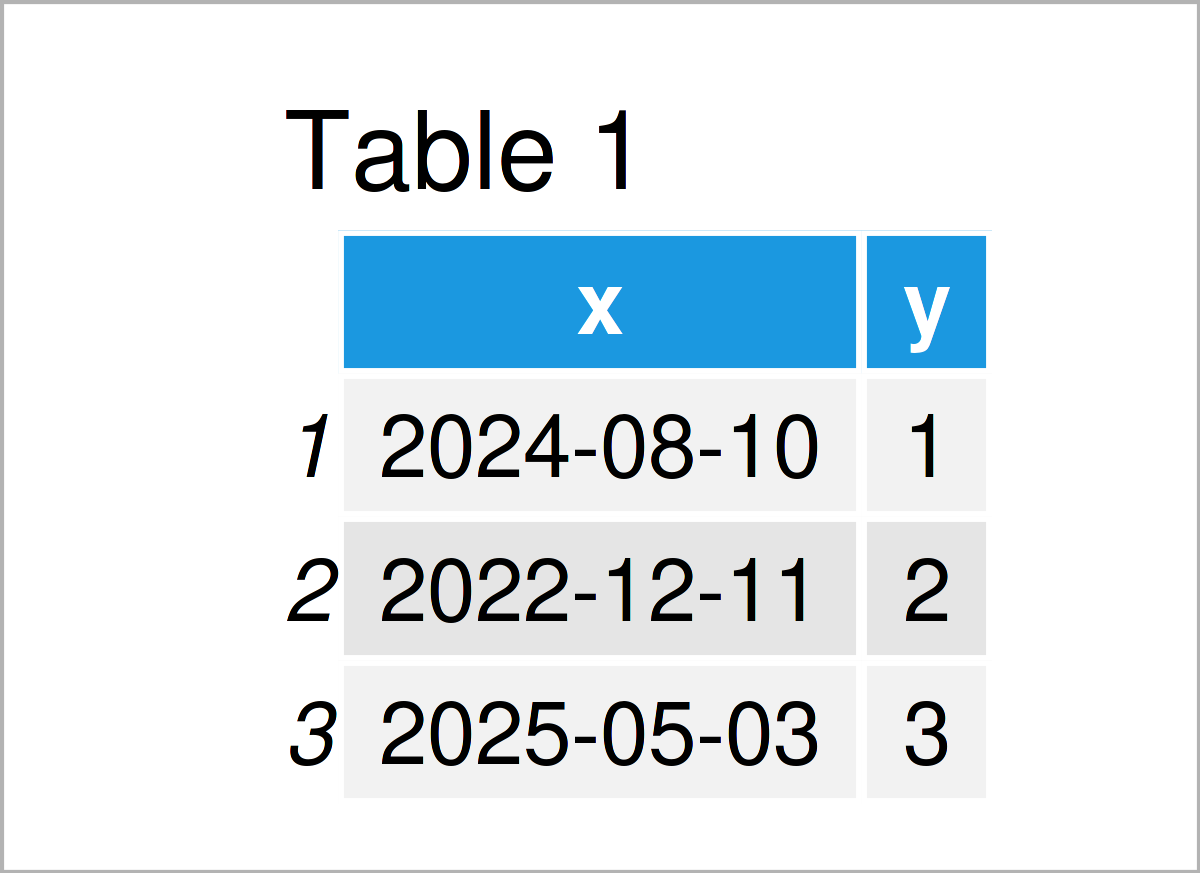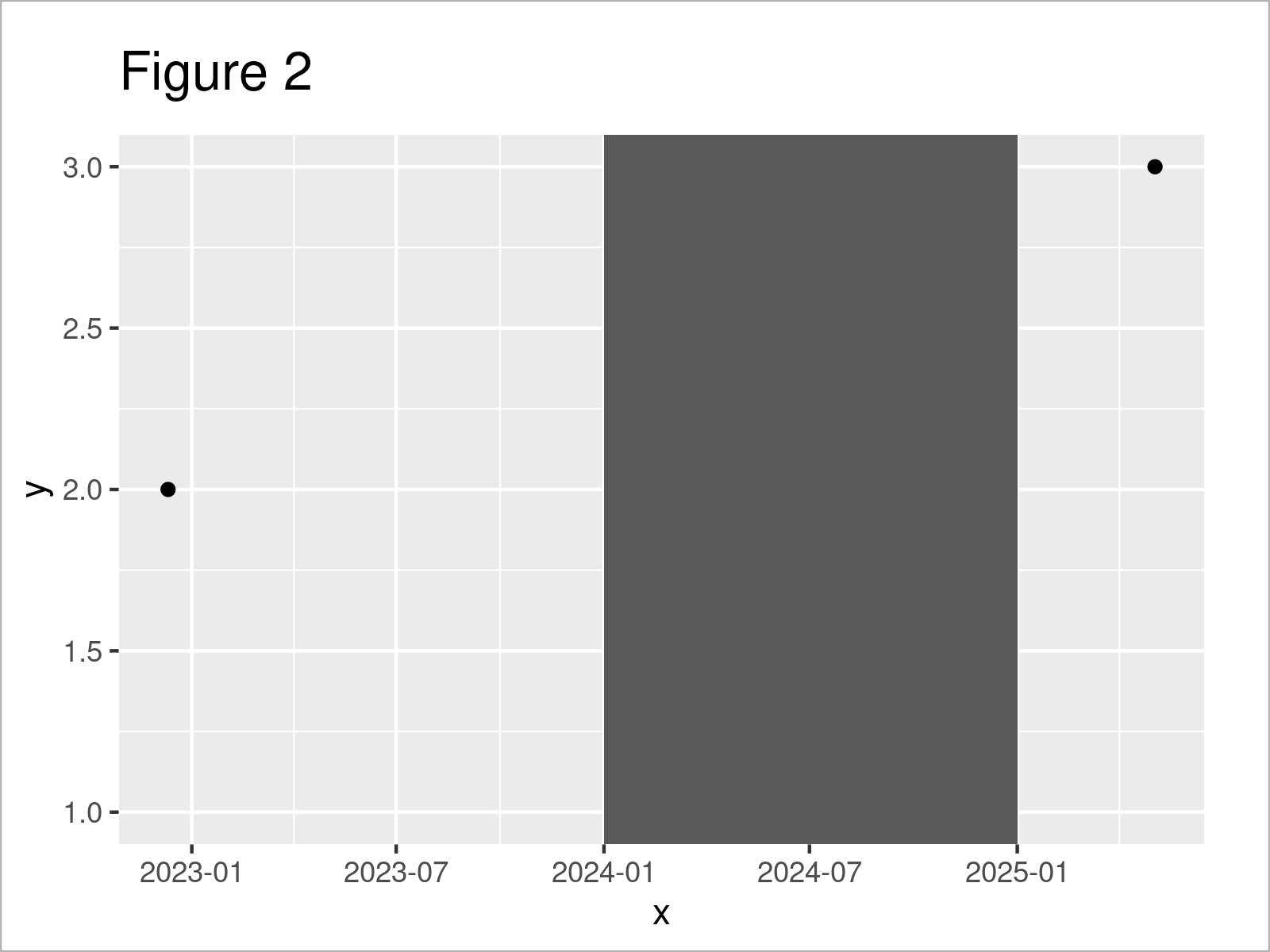# ggplot2 Error in R: Invalid input: date_trans works with objects of class Date only (2 Examples)

On this page, I’ll show how to deal with the “Error: Invalid input: date_trans works with objects of class Date only” in the R programming language.

Let’s dig in.

## Example Data, Add-On Packages & Basic Graph

The following data is used as basement for this R tutorial:

```data <- data.frame(x = as.Date(c("10-08-2024",    # Create example data
"11-12-2022",
"03-05-2025"),
format = "%d-%m-%Y"),
y = 1:3)
data                                              # Print example data```Table 1 shows that our example data is constructed of three rows and two columns.

For the following R code, we’ll also need to install and load the ggplot2 package:

```install.packages("ggplot2")                       # Install ggplot2 package

Next, we can draw our data:

```ggp <- ggplot(data, aes(x, y)) +                  # Create ggplot2 scatterplot with dates
geom_point()
ggp                                               # Draw ggplot2 scatterplot```After running the previous R programming syntax the ggplot2 time series scatterplot with dates on the x-axis shown in Figure 1 has been created.

## Example 1: Reproduce the Error: Invalid input: date_trans works with objects of class Date only

The following R programming code shows how to replicate the ggplot2 “Error: Invalid input: date_trans works with objects of class Date only”.

Let’s assume that we want to add a rectangle using the geom_rect function to our plot. Then, we might try to use the following R code:

```ggp +                                             # Replicating the error
geom_rect(data = data,
aes(xmin = "01-01-2024",
xmax = "01-01-2025",
ymin = -Inf,
ymax = Inf))
# Error: Invalid input: date_trans works with objects of class Date only```

Unfortunately, the RStudio console returns the “Error: Invalid input: date_trans works with objects of class Date only” after executing the previous syntax.

The reason for this is that we have not specified the dates within geom_rect properly.

Let’s solve this problem!

## Example 2: Debug the Error: Invalid input: date_trans works with objects of class Date only

The following R syntax explains how to handle the “Error: Invalid input: date_trans works with objects of class Date only”.

For this, we have to apply the as.Date function to the dates within geom_rect. Depending on the format of your dates, you also have to specify the format argument accordingly:

```ggp +                                             # Fixing the error
geom_rect(data = data,
aes(xmin = as.Date("01-01-2024", format = "%d-%m-%Y"),
xmax = as.Date("01-01-2025", format = "%d-%m-%Y"),
ymin = -Inf,
ymax = Inf))```As you can see in Figure 2, we have created a ggplot2 time series plot with a rectangle element. No errors anymore!

## Video & Further Resources

In case you are keen to learn more about the ggplot2 package and data visualization in R, please have a look at the following video tutorial, where I give a detailed introduction with different examples:

Please accept YouTube cookies to play this video. By accepting you will be accessing content from YouTube, a service provided by an external third party.If you accept this notice, your choice will be saved and the page will refresh.

Also, you may want to have a look at the related tutorials on https://www.statisticsglobe.com/.

In summary: In this tutorial, you have learned how to avoid the “Error: Invalid input: date_trans works with objects of class Date only” in R. Kindly let me know in the comments below, if you have additional questions and/or comments.

Subscribe to the Statistics Globe Newsletter